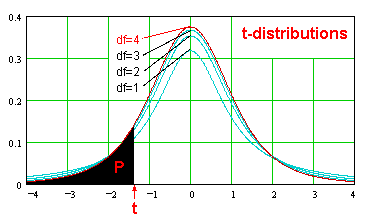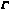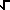Home    |    Teacher    |    Parents    |    Glossary    |    About UsEmail this page to a friendResources· Cool Tools · Formulas & Tables · References · Test Preparation · Study Tips · Wonders of MathSearcht-distributions/b> (Math | Distributions | t-Distributions)The t-distribution, with n degrees of freedom, is given by the equation:

f(t) = [((n + 1)/2) (1 + x^2 / n)^(-n/2 - 1/2) ] / [(n/2)(PI n) ] (See also Gamma Function.)